# Polynomial Function

## Polynomial Function

Polynomial Function is defined as the real valued function $f : R \rightarrow R$ if for each $x \in R$ $y = f(x)=a_nx^n+a_{n-1}x^{n-1}+a_{n-2}x^{n-2}+.....+a_1x+a_0$
Where $a_n,a_{n-1},....,a_1,a_0$ are constant and real numbers and n is a non-negative integer
Some important points to remember
• an,an-1,...,a,a0 are called the coefficients for xn,xn-1 ,..,x,x0
• n is called the degree of the polynomial function
• A Constant Function is the polynomial function with zero degree
• n is a non-negative integer.It can not be fraction also
Example of Polynomial functions
$3x^2 + 4x+6$
$9x^3 -4x^2 +1$
$4x +1$
$4x^2 + \sqrt {2}$

The below are not polynomial functions
$x^{-4} + 1$
$\sqrt {x} + 2x +1$
$x^{2/3} +1$

### Types of Polynomial function based on degree

 Type of Polynomial function Example Degree Constant Function $1$ n=0 Linear Function $4x +1$ n=1 Quadratic function $x^2 +4x +1$ n=2 Cubic Function $3x^3 -4x^2+x +11$ n=3 Quartic Function $x^4 +2x^3 +4x^2+x +1$ n=4

Function f(x) =0 is also an polynomial function with undefined degree

## Domain and Range of the Polynomial Function

Domain = R
Range is dependent on the type of polynomial function.
For Linear function ,Range is R
For constant function Range is {c}
For Quadratic function like ${x^2 +1}$ , Range is $[1,\infty)$
We can apply the below steps to find the Range of Quadratic Functions easily
Step 1: Quadratic Function will be of type
$y=ax^2 + bx + c$
Step 2: Quadratic function will have minimum value or maximum value depending on the coefficent a
if a > 0 , It will have minimum value
if a < 0, it will have maximum value
Step 3: The minimum or maximum values can be found using
a. First find the value of x where it will be minimum or maximum
$x_0 = - \frac {b}{2a}$
b. Now minimum or maximum value can be found using
$y_0 = f(x_0)$
Step 4: Range will be given as
if a > 0 , Range will be $[y_0,\infty)$
if a < 0 , Range will be $(-\infty,y_0]$
For Cubic functions ,Range is R

## Graph of the Polynomial Function

The graph of the Polynomial function depends on the type of polynomial functions.

### For constant and Linear function

It is always straight line for constant and Linear Function
Here are the graph for function
$f(x)=3$ ( Constant function)
$f(x)=x+2$(Linear Function)
$f(x)=3x+1$(Linear Function)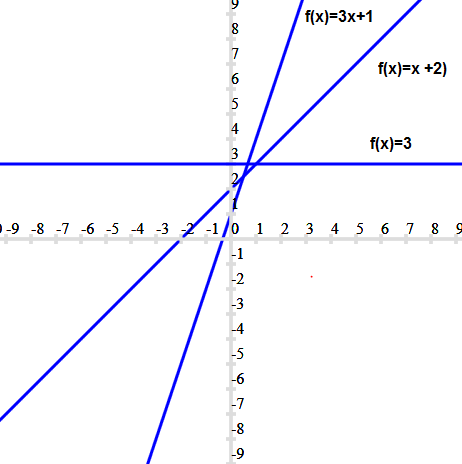For Quadratic function, the graph is a parabolic graph. Lets see few example

1. $f(x) =2x^2$ , $h(x) =5x^2$ , $g(x) =10x^2$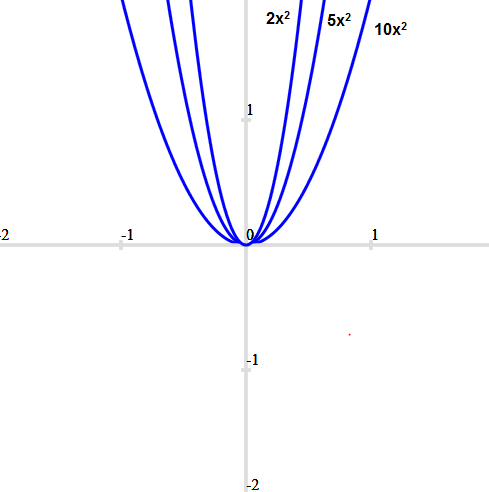We can see the graph is a upward parabola and as we increase the coefficient of $x^2$,the graph is stretched vertically

2. $f(x) =-2x^2$ , $h(x) =-5x^2$ , $g(x) =-10x^2$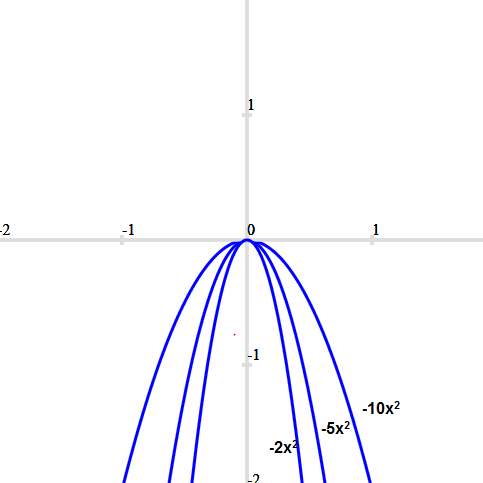We can see the graph is a downward parabola and as we increase the coefficient of $x^2$,the graph is stretched vertically

3. $f(x) = x^2 + 2x +1$ , $g(x) = x^2 + 4x +1$ ,$h(x) =x^2 +6x +1$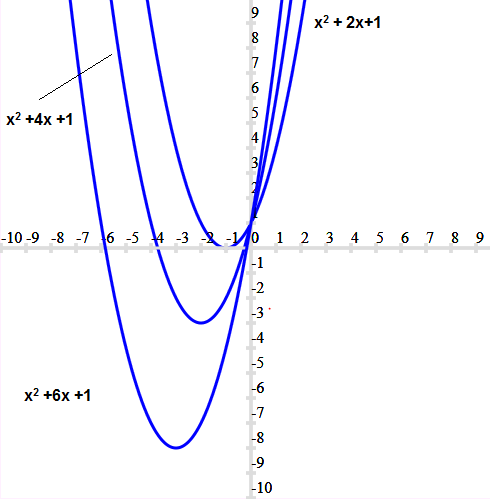We can see the graph is a upward parabola and with increase in coefficient of x ,it drifts downwards on the left

4. $f(x) =x^2 +1$ and $h(x) =-x^2 -1$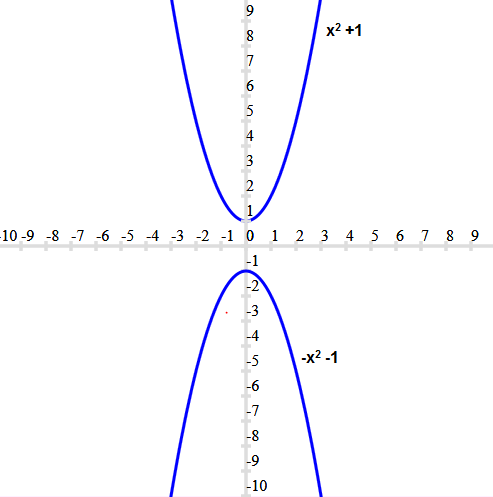Here since their are no real roots of the given quadratic function , the graph is not touching the x-axis. First graph is upward above x-axis and second graph is downward below x-axis
So , the intersection of parabola graph depends on the real roots of the function

5.$f(x) =x^2 -5x +6$ and $h(x) =-x^2 +7x -12$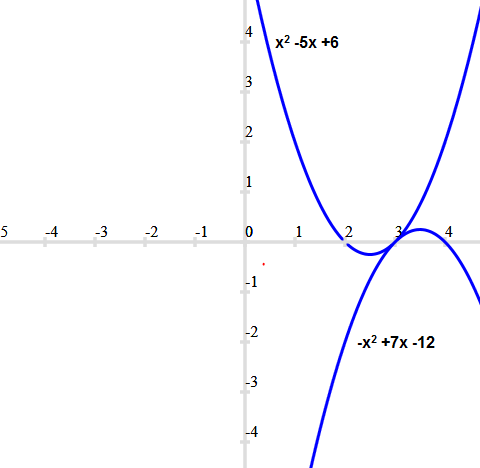Here since their are real roots of the given quadratic function ,the graph is intersecting the the x-axis.

### For Cubic function

Lets see few example of the cubic function
1. $f(x) =x^3$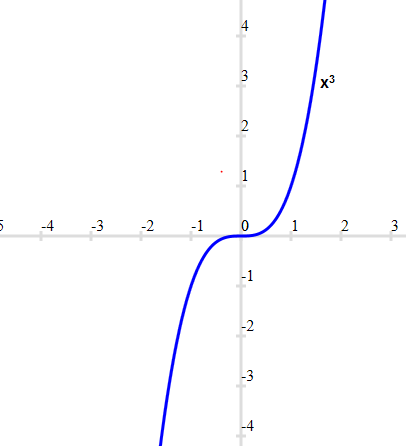We can see it extends in both upward and downward direction

2. $f(x) = x^3 + 1$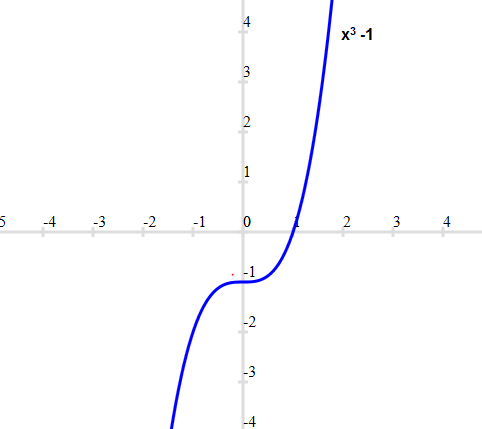Here is the cubic function with one real root

3.$f(x) = x^3 -3x^2 +3x -1= (x-1)^3$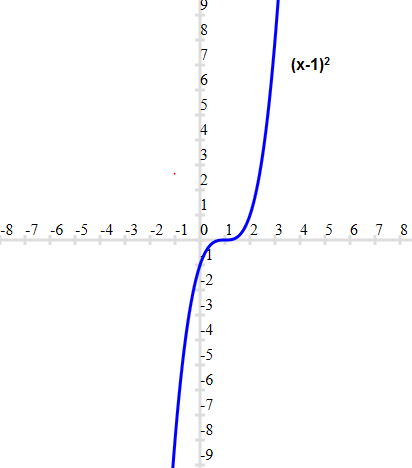Here is the cubic function with three equal real root

4.$f(x) = x^3+12x^2+39x+28=(x+1)(x+4)(x+7)$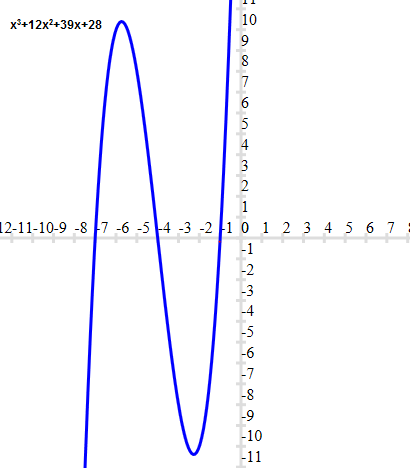Here is the cubic function with three different real root.

### For Quartic function

1. $f(x) =x^4 -5x^2 +4 =(x-1)(x-2)(x+1)(x+2)$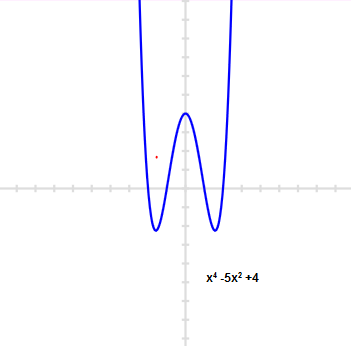This is a quartic function and it has four real roots .

Some important points on Polynomial functions graphs

1. The turning point in a graph is defined as the points from where graph from upward to downward or downward to upward. The turning points in the graph is always less or equal to (n-1) of the polynomial function.So a quartic function has maximum 3 turning points in the graph.A quadratic equation has maximum one turning point. A Cubic equation has maximum 2 turns. You can validate these in above given example graph also

2. The zero's or root of the polynomial function are point at which graph intersect x-axis , i,e the point where the value of y=0. The real roots of the polynomial function is always less or equal to the degree n of the polynomial.

## How to Solve Polynomial functions

Solving Polynomial functions means finding roots ,domain , range of the polynomial functions. Here are few links which will give good description about finding zeros
How to Factor Polynomials
We have already discussed above on how to find domain and range also

## Solved examples of Polynomial Functions

1. Find the domain of the below function?
a. $y =x^2 +5$
b. $y = x^3$
c. $y=x^2 -7x +5$
Solution

a. Clearly it is defined for all $x \in R$
b. Clearly it is defined for all $x \in R$
c. Clearly it is defined for all $x \in R$

2. Find the domain and range of function
$y=3x^2+12x−12$
Solution
We have ,
$y=3x^2+12x−12$
Domain of y
Clearly it is defined for all $x \in R$
Range of y
Comparing it to $y=ax^2 + bx +c$
Then a=3, b=12 c=-12
Now since a> 0,then it will have minimum value
Now Minimum value will occur at
$x_0 = - \frac {b}{2a} = -2$
So minimum value is
$y_0= f(x_0) = 3 \times (-2)^2 + 12 (-2) - 12= -24$
So range is $[-24, \infty)$

3. Let $f : R \rightarrow R$ , $f(x) = x^2 + 2[x] -1$ for each $x \in R$
Find the values of f(x) at x= 1.2 ,-.5 ,-2.1
Solution
$f(x) = x^2 + 2[x] -1$
$f(1.2) = (1.2)^2 + 2[1.2] -1 =1.44 +2-1=2.44$
$f(-.5) = (-.5)^2 + 2[-.5] -1 =.25 -2-1=-2.75$
$f(-2.1) = (-2.1)^2 + 2[-2.1] -1 =4.41 -6-1=-2.59$

### Quiz Time

Question 1.
Find the domain of the function $f(x)= \sqrt {x^2 -1}$
A. R
B. ${-\infty,-] \cup [1,\infty}$
C. R -{1}
D. R -{1,-1}
Question 2.
Find the range of the function $y=-x^2 -2x -4$
A. $[-2,\infty)$
B. $[3,\infty)$
C. $[-2,-3) \cup [2,3)$
D. $(-\infty, -3]$
Question 3.
if $f(x) =[x]^2 +[x] +2$,then which of them is incorrect
A. f(1)=4
B. f(.75)=2
C. f(-.50)=2
D. f(0) =3
Question 4.
Value of the expression [{x}] + {[y]} is
A. 1
B. 0
C. 2
D. Cannot be determined
Question 5.
The value {-.75} where {.} is the fractional part is
A. -.75
B. .25
C. 0
D. None of these
Question 6.
The domain of the function f given by $f (x) = \sqrt {16 -x^2}$ is
A. [-4,4]
B. {-4,4}
C. [0,4]
D. [-4,0]